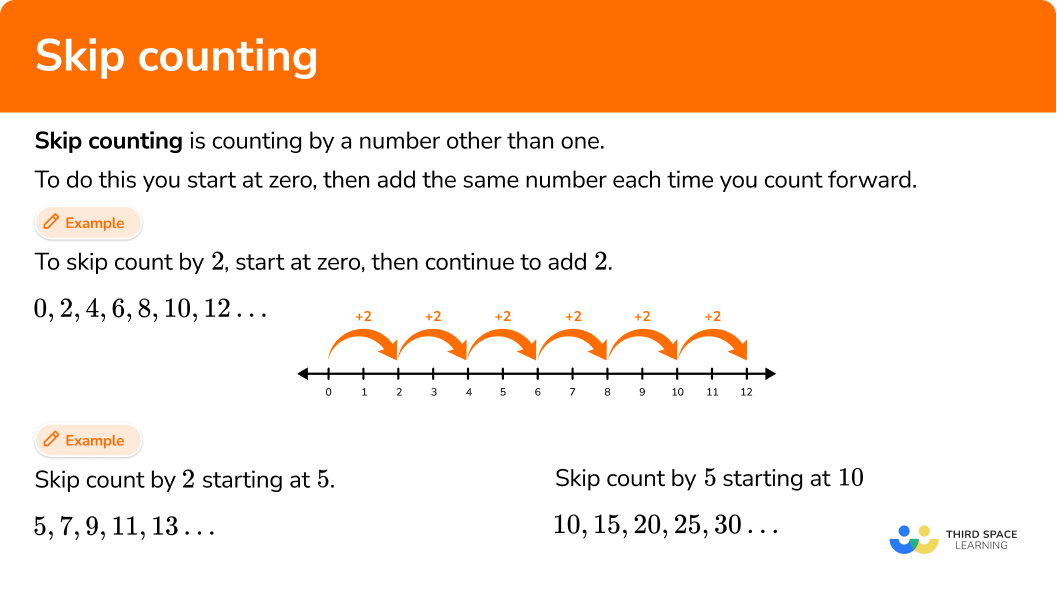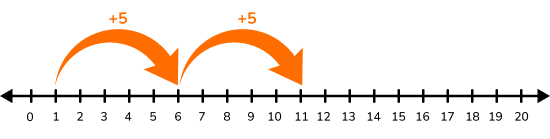# Skip counting

Here you will learn about skip counting, including skip counting by twos, fives, tens, and hundreds, and skip counting using a number line.

Students will first learn about skip counting as part of counting and cardinality in Kindergarten and will extend their knowledge as part of number and operations in base ten in 2nd grade.

## What is skip counting?

Skip counting is counting by a number other than one.

To do this, you start at zero and add the same number each time you count forward.

For example, to skip count by 2, start at zero, then continue to add 2.

0, 2, 4, 6, 8, 10, 12…

You can show skip counting using a number line.

You can skip count starting at any number.

For example,

Skip count by 2 starting at 5.

5, 7, 9, 11, 13…

Skip count by 5 starting at 10.

10, 15, 20, 25, 30…

### What is skip counting?## Common Core State Standards

How does this relate to Kindergarten math and 2nd grade math?

• Kindergarten – Counting and Cardinality (K.CC.1)
Count to 100 by ones and by tens.

• 2nd grade – Number and Operations in Base Ten (2.NBT.2)
Count within 1000; skip-count by 5s, \, 10s, and 100s.

## How to skip count

In order to skip count:

1. Find the starting number. If no starting number is mentioned, start at zero.
2. Identify the number you will be skip counting by and add/subtract it to the starting number.
3. Continue to add the number to the previous number in the sequence in order to find the next number.

## Skip counting examples

### Example 1: skip count by 5

Skip count by 5 up to 50.

1. Find the starting number. If no starting number is mentioned, start at zero.

Since no starting number was mentioned, start at zero.

0,

2Identify the number you will be skip counting by and add/subtract it to the starting number.

You are skip counting by 5, so you need to add 5 to the starting number of zero.

0 + 5 = 5

0, 5,

3Continue to add the number to the previous number in the sequence in order to find the next number.

5 + 5 = 10

10 + 5 = 15…

If you continue this to 50, your sequence will be,

0, 5, 10, 15, 20, 25, 30, 35, 40, 45, 50

### Example 2: skip count by 100

Skip count by 100 up to 1,000.

Find the starting number. If no starting number is mentioned, start at zero.

Identify the number you will be skip counting by and add/subtract it to the starting number.

Continue to add the number to the previous number in the sequence in order to find the next number.

### Example 3: skip counting odd numbers

Skip count by 2, starting at 1. Stop at 21.

Find the starting number. If no starting number is mentioned, start at zero.

Identify the number you will be skip counting by and add/subtract it to the starting number.

Continue to add the number to the previous number in the sequence in order to find the next number.

### Example 4: skip counting on a number line

Using a number line, skip count by 5 starting at 2.

Find the starting number. If no starting number is mentioned, start at zero.

Identify the number you will be skip counting by and add/subtract it to the starting number.

Continue to add the number to the previous number in the sequence in order to find the next number.

### Example 5: skip counting backwards

Fill in each blank by skip counting backwards by 10 starting at 74.

Find the starting number. If no starting number is mentioned, start at zero.

Identify the number you will be skip counting by and add/subtract it to the starting number.

Continue to add the number to the previous number in the sequence in order to find the next number.

### Example 6: skip counting word problem

Jamie has 6 baskets. Each basket has 3 apples. Skip count by 3 to find out how many apples Jamie has altogether.

Find the starting number. If no starting number is mentioned, start at zero.

Identify the number you will be skip counting by and add/subtract it to the starting number.

Continue to add the number to the previous number in the sequence in order to find the next number.

### Teaching tips for skip counting

• Incorporate skip counting practice into math centers by providing students with skip counting activities and math games involving skip counting.

• Provide students with skip counting practice by having a variety of printable skip counting worksheets available for students to use in math centers or for morning work.

• Students can also use number flash cards for a quick and easy math activity – students can choose a number to skip count by and find the flash cards that create the sequence.

• Ask students to create skip counting posters. These can include the most common numbers used for skip counting (2, 5, 10, 100) or all numbers up to 10.

• Give students a challenge by asking them to skip count backward. This will require them to use subtraction instead of addition.

• Ask students what number patterns they notice when skip counting. This will help set the foundation for their understanding of number patterns and multiplication tables in later grades.

• For a fun, whole-class skip counting activity, ask students to stand in a circle and give them a number from 2-10 or 100. The first student starts with that number, then the students go around the circle skip counting by saying the next number aloud until each student says a number.

### Easy mistakes to make

• Starting at the wrong number
Students may think skip counting always begins at zero. However, some questions will give students a different starting number.

• Thinking that skip counting by certain numbers will always show the same pattern
Students may have preconceived notions about skip counting by common numbers such as 2 and 5. If students are skip counting by 2s, they may automatically think they are skip counting even numbers starting with number 2.
However, if the question asks them to skip count by 2 starting at 1, the sequence will include 1, 3, 5, 7, 9… and so on. Not only will this not include the number 2, but the sequence will only include odd numbers.

### Practice skip counting questions

1. Adrian skip counted by 3s. What is the missing number?

0, 3, 6, 9, ___, 15, 18, 21…

1231114Since Adrian is skip counting by 3s and the missing number comes after 9, you can add 3 to 9 to find the missing number of 12.

2. Lily skip counted by 2s starting at 46. What is the missing number?

46, 48, ___, 52, 54, 56…

49514650Since Lily is skip counting by 2s and the missing number comes after 48, you can add 2 to 48 to find the missing number of 50.

3. Maria used a number line to skip count by 5s starting at 1. What is the next number she should jump to?15201610Since the last number the number line points to is 11, Maria would need to add 5 to 11. This means the next number she should jump to is 16.

4. Which sequence of numbers shows skip counting by 4?

4, 6, 8, 10, 12…0, 4, 9, 13, 18…6, 10, 14, 18, 22…1, 2, 3, 4…Since you are skip counting by 4s, you should be able to add 4 to each number to get to the next number in the sequence. The number “4” does not need to be in the answer.

5. Summer decided to challenge herself and skip count by sevens! Her sequence of numbers is shown below. What was her starting number?

___, 33, 40, 47, 54…

7276126Since Summer is skip counting by sevens, you should be able to add 7 to each number to get to the next number in the sequence.

However, since you are finding the starting number, instead of adding seven, you can subtract seven from the second number in the sequence. If you subtract seven from 33, you get Summer’s starting number of 26.

6. Miles skip counted by 10s to place marbles into 8 jars. He placed 10 marbles in the first jar, 20 marbles in the second jar, and so on until all jars were filled.

How many marbles were in the 6th jar?

10605080If you count by 10s, the 6th number will be 60.

10, 20, 30, 40, 50, 60

## Skip counting FAQs

How is skip counting related to multiplication tables?

Skip counting sets the foundation in 2 nd grade for students to begin learning multiplication facts in 3 rd grade. For example, to solve 5 \times 3, students can skip count by fives 3 times: 5, 10, 15.

What are the most common skip counting numbers?

The most common skip counting numbers are 2, 5, 10, and 100. However, many students will also practice skip counting by the remaining numbers under 10.

Why is skip counting important?

Skip counting is important because it strengthens number sense and place value understanding for young learners. It also provides a strong foundation for future math skills, such as multiplication tables, number patterns, and even fractions and decimals.

## Still stuck?

At Third Space Learning, we specialize in helping teachers and school leaders to provide personalized math support for more of their students through high-quality, online one-on-one math tutoring delivered by subject experts.

Each week, our tutors support thousands of students who are at risk of not meeting their grade-level expectations, and help accelerate their progress and boost their confidence.